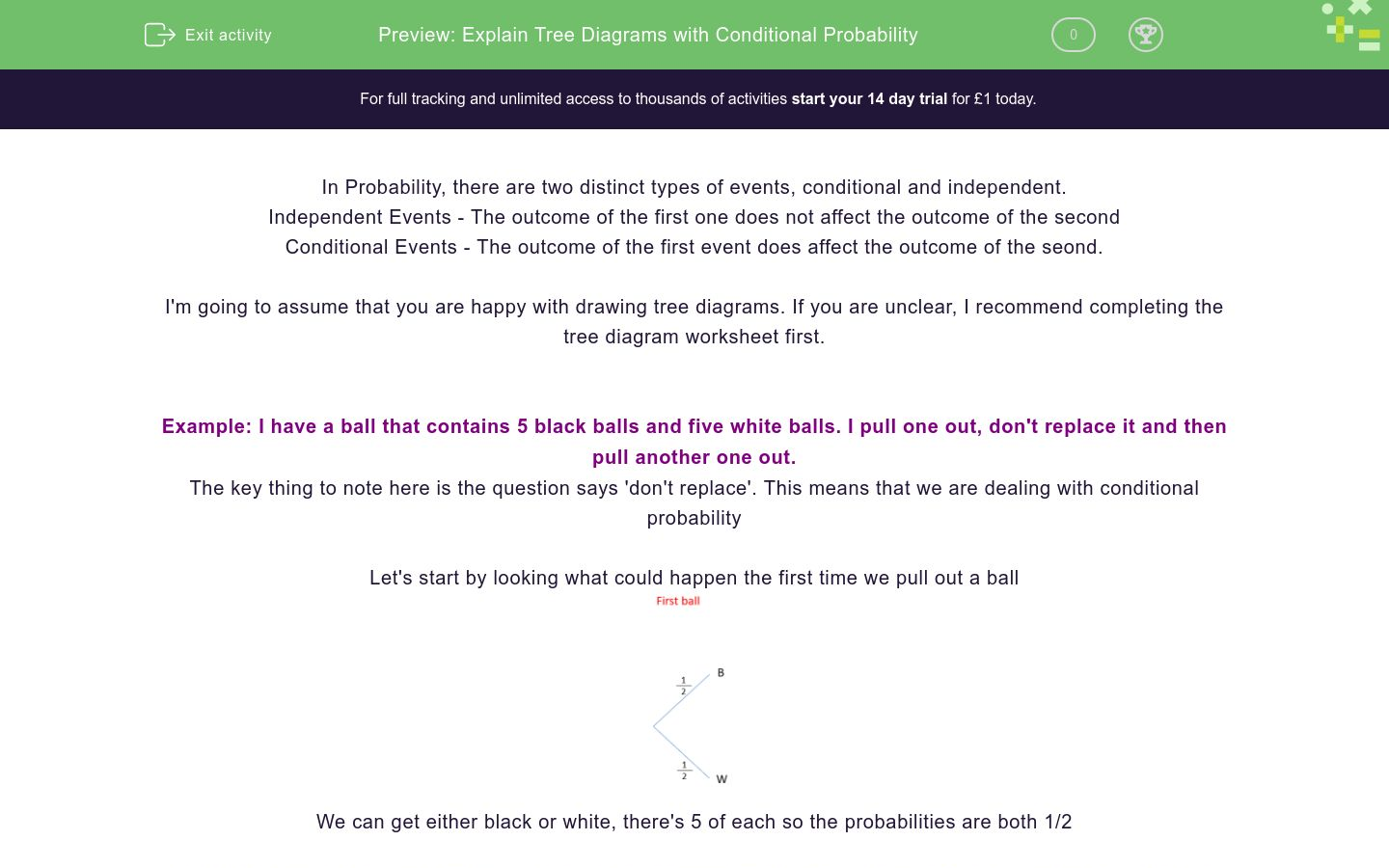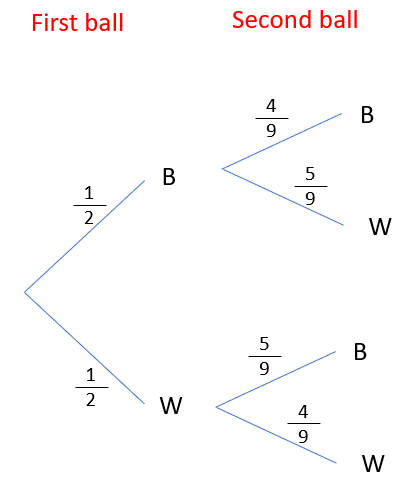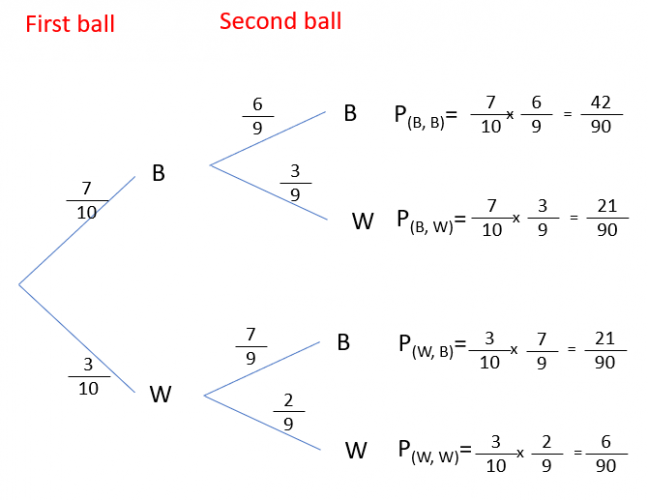# Explain Tree Diagrams with Conditional Probability

In this worksheet, students practise creating and using tree diagrams involving conditional probability.Key stage:  KS 4

GCSE Subjects:   Maths

GCSE Boards:   AQA, Eduqas, Pearson Edexcel, OCR

Curriculum topic:   Probability

Curriculum subtopic:   Probability, Combined Events and Probability Diagrams

Difficulty level:### QUESTION 1 of 10

In Probability, there are two distinct types of events, conditional and independent.

Independent Events - The outcome of the first one does not affect the outcome of the second

Conditional Events - The outcome of the first event does affect the outcome of the seond.

I'm going to assume that you are happy with drawing tree diagrams. If you are unclear, I recommend completing the tree diagram worksheet first.

Example: I have a ball that contains 5 black balls and five white balls. I pull one out, don't replace it and then pull another one out.

The key thing to note here is the question says 'don't replace'. This means that we are dealing with conditional probability

Let's start by looking what could happen the first time we pull out a ballWe can get either black or white, there's 5 of each so the probabilities are both 1/2

Let's now look at the second branches. If I pulled out a black first time, I would now have 4 black and 5 white balls.

This is going to change the probabilitiesI can now do the same for white first. If I pulled out a white first, I have 5 black balls and 4 white balls.From here, I complete this the same way I would a normal probability tree.You could if you wanted to, cancel these fractions down now, I would however recommend leaving them. In a moment we might have to add them together and if they all have the same denominator it'll be easier.

We can always cancel down at the end.

How do we use this?

Tree diagrams are all about finding probabilities for combined events. Lets look at an example.

What is the probability that I pull out at least one black.

There are three ways this can happen. BB, BW and WB

All we have to do is find the ones on the tree that show this (They're the top 3) and add them together.

4/18 + 5/18 + 5/18 = 14/18 = 7/9

Complete the following sentence.

A bag contains 7 black and 3 white balls. I withdraw w without replacement.

For the tree diagram...Find the values of A, B, C and D

{HINT - You will find it easier for this, and the questions that are next, if you draw this out on paper first}

 Probability A B C D

A bag contains 7 black and 3 white balls. I withdraw w without replacement.What is the probability I will get two black balls?

42/100

42/90

7/15

A bag contains 7 black and 3 white balls. I withdraw w without replacement.What is the probability I will get at least one white?

I have 15 cards, 10 have A on them and 5 have B. I pick two at random.

For the tree diagram...Find the values of A, B, C and D

{HINT - You will find it easier for this, and the questions that are next, if you draw this out on paper first}

 Probability A B C D

I have 15 cards, 10 have A on them and 5 have B. I pick two at random.

For the tree diagram...What is the probability I will have get B twice?

9/21

2/21

I have 15 cards, 10 have A on them and 5 have B. I pick two at random.

For the tree diagram...What is the probability I will get A at least once

In a carton of 6 eggs, 5 are brown and 1 white. If i take out two eggs at random.

Complete the tree diagram...Find the values of A, B, C and D

{HINT - You will find it easier for this, and the questions that are next, if you draw this out on paper first}

 Probability A B C D

In a carton of 6 eggs, 5 are brown and 1 white. If i take out two eggs at random.What is the probability that the two eggs I pick will not be the same.

1/6

1/3

In a carton of 6 eggs, 5 are brown and 1 white. If i take out two eggs at random.What is the probability that the two eggs I pick will be the same.

• Question 1

Complete the following sentence.

EDDIE SAYS
This is the crux of conditional probability. They do affect each other and the probabilities will change.
• Question 2

A bag contains 7 black and 3 white balls. I withdraw w without replacement.

For the tree diagram...Find the values of A, B, C and D

{HINT - You will find it easier for this, and the questions that are next, if you draw this out on paper first}

 Probability A B C D
EDDIE SAYS
Did you get that the probabilities for the second branch were out of 9? If I get a black first, I have 6 black and 3 white left If I get white first, I have 7 black and 2 white left All you then need to do is multiply through as normal.
• Question 3

A bag contains 7 black and 3 white balls. I withdraw w without replacement.What is the probability I will get two black balls?

42/90
7/15
EDDIE SAYS
Be careful with your answers. Did you notice there were two answers you could have used? One was simplified and one wasn't but they were both correct.
• Question 4

A bag contains 7 black and 3 white balls. I withdraw w without replacement.What is the probability I will get at least one white?

8/15
EDDIE SAYS
You need to start this question by asking how this could happen. There are 3 ways this could happen. (BW WB WW) All we have to do is add the three probabilities for these events together.
• Question 5

I have 15 cards, 10 have A on them and 5 have B. I pick two at random.

For the tree diagram...Find the values of A, B, C and D

{HINT - You will find it easier for this, and the questions that are next, if you draw this out on paper first}

 Probability A B C D
EDDIE SAYS
Did you get that the probabilities for the second branch were out of 14? If I get a A first, I have 9 As left and 5 Bs If I get B first, I have 10 As left and 4 Bs
• Question 6

I have 15 cards, 10 have A on them and 5 have B. I pick two at random.

For the tree diagram...What is the probability I will have get B twice?

2/21
EDDIE SAYS
People often make mistakes here. To find the final outcomes from a tree diagram, we multiply the probabilities of each event. We need to find the probability for BB. We can see this is the bottom of the four outcomes. All we need to do is cancel.
• Question 7

I have 15 cards, 10 have A on them and 5 have B. I pick two at random.

For the tree diagram...What is the probability I will get A at least once

19/21
EDDIE SAYS
You need to start this question by asking how this could happen. There is only one way this couldn't happen so I add the probabilities for the rest and cancel down.
• Question 8

In a carton of 6 eggs, 5 are brown and 1 white. If i take out two eggs at random.

Complete the tree diagram...Find the values of A, B, C and D

{HINT - You will find it easier for this, and the questions that are next, if you draw this out on paper first}

 Probability A B C D
EDDIE SAYS
This is pretty much the same as the others. But did you get the probability of WW as 0? Remember 0 means impossible and we only have one white egg.
• Question 9

In a carton of 6 eggs, 5 are brown and 1 white. If i take out two eggs at random.What is the probability that the two eggs I pick will not be the same.

1/3
EDDIE SAYS
Think about how this can happen. We could either get BW or WB. 1/6 + 1/6 = 2/6 = 1/3
• Question 10

In a carton of 6 eggs, 5 are brown and 1 white. If i take out two eggs at random.What is the probability that the two eggs I pick will be the same.

2/3
EDDIE SAYS
You need to start this question by asking how this could happen. We already know that there is no way we can get two whites, so the only way to get this is to get 2 brown eggs.
---- OR ----

Sign up for a £1 trial so you can track and measure your child's progress on this activity.

### What is EdPlace?

We're your National Curriculum aligned online education content provider helping each child succeed in English, maths and science from year 1 to GCSE. With an EdPlace account you’ll be able to track and measure progress, helping each child achieve their best. We build confidence and attainment by personalising each child’s learning at a level that suits them.

Get started